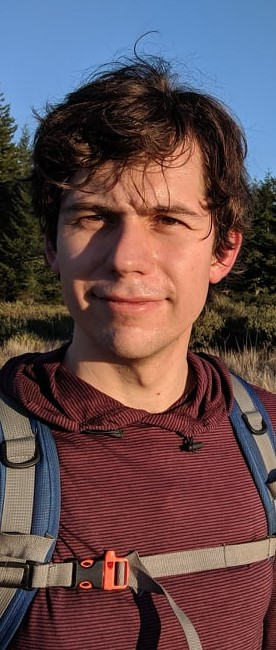# Semon RezchikovI am a Veblen Research Instructor and NSF Postdoctoral Fellow in the Mathematics Department at Princeton University and the Institute of Advanced Study.

As a mathematician, I am interested in symplectic geometry, which is the geometry of phase spaces of physical systems, or more generally the geometry of the principle of least action. While the importance of symplectic geometry was known since Lagrange, the subject remained underdeveloped until the 80s, when Gromov's introduction of pseudoholomorphic curve theory, a nonlinear version of complex analysis, led to a continual transformation of the field. It turns out that the pseudoholomorphic curves in a symplectic manifold are organized into elaborate algebraic structures, which can be interpreted as a supersymmetric field theory which mirrors simpler structures related to polynomials, and incidentally gives rise to a quantum generalization of topology. Remarkably, these exotic connections between disparate areas of mathematics and physics can be used to solve concrete problems in chaotic dynamics and even children's problems about drawing rectangles inside curves.

My research has focused on foundational aspects of the invariants built from pseudoholomorphic curves, as well as on applications of these "quantum" modifications of topology to dynamical systems theory. I have also recently been working on complexifying symplectic geometry, which turns out to be connected to a quaternionic version of pseudoholomorphic curve theory and to the mysterious subject of 3D mirror symmetry.

Beyond that, I am interested in applications of mathematics. The boundaries between physics, computer science, statistics, and mathematics have never been clear, and their interaction between remains a source of new ideas for me. In particular, I am working on rigorous ways to implement ideas from the renormalization group in machine-learning based models of physical systems.

In the past, you may have seen me at

My favorite book is Edmund Spenser's Faerie Queene.

### Contact

Email: semonr at princeton dot edu

Snail mail:
Department of Mathematics
Princeton University
Fine Hall, Washington Rd

### Mathematics

• Holomorphic Floer Theory and the Fueter Equation
(joint with Alexander Doan.) This paper outlines the structure of the Fueter 2-category, a 2-category assigned to a hyperkahler manifold M which categorifies the Fukaya category of M. We introduce new maximum principles for Fueter maps and prove an adiabatic limit theorem which categorifies and complexifies Floer's theorem on pseudoholomorphic curves in cotangent bundles. The Fueter 2-category is the 2-category of boundary conditions of the A-twist of the 3D N=4 sigma nodel, and as such sits at the intersection of several different fields of mathematics and physics which I am excited to explore.
• Integral Arnol'd Conjecture
This paper constructs global charts for Hamiltonian Floer homology following Abouzaid-McLean-Smith. As a byproduct we prove the Integral Arnol'd conjecture using the Fukaya-Ono-Parker perturbations of Bai-Xu. I see global charts as a flexible tool to be used in future applications.
• Rational Quantum Cohomology of Steenrod Uniruled Manifolds
This paper closes the gap between the usual formulation of the Chance-McDuff conjecture, which states that the existence of a Hamiltonian diffeomorphism with finitely many periodic points forces nontrivial rational Gromov-Witten invariants, and recent results that use methods in equivariant Floer theory to prove variants of this conjecture via equivariant, positive-characteristic Gromov-Witten invariants. The proof is a combination of a certain amount of `symplectic birational geometry' and a calculation using recently established properties of the quantum Steenrod algebra.
• Generalizations of Hodge-de-Rham degeneration for Fukaya categories, draft version.
I explain why certain conjectures of Kontsevich about cyclic homology of categories always hold for Fukaya categories, even though Efimov demonstrated that they do not hold in general. The proof gives a TQFT interpretation of the conjectures, and a conceptual perspective on why they turned out to be in error.
• Floer homology via Twisted Loop Spaces, submitted.
This is a paper about signs. I explain how to extract an integral variant of Lagrangian Floer homology from pseudoholomorphic curve moduli spaces which may not be orientable. This anwers a question of Witten and lets one prove stronger Lagrangian self-intersection bounds.

Applications of Mathematics

### Teaching

In 2020, I taught Calculus II (UN1102) at Columbia University. In 2019 I co-organized with Kyler Siegel a Columbia REU program for undergraduate students. The students used numerical optimization methods, tools from symplectic integration, and Haim-Kislev's recent work to find new symplectic embeddings. I have also TAed for graduate and undergraduate courses.